## Calculate median in Excel: with two practical examples

The median is a form of measure of central tendency that represents the middle value in a sorted list of numbers. In Excel, finding the median is straightforward, thanks to the built-in MEDIAN function. Unlike the average, the median is not skewed by extremely high or low values, making it a robust indicator of the data's center.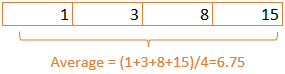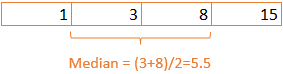##### Introduction to MEDIAN function
``MEDIAN(number1, [number2],…)``
• This function sorts a range of numbers (number1, number2,…) and returns the one that falls in the middle. For an odd number of observations, the median is the number that splits the dataset into two halves. For an even number of observations, Excel averages the two middle numbers.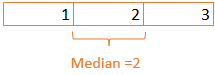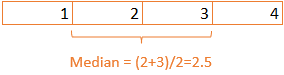• The MEDIAN function in Excel excludes text, logical values, and blank cells but includes zeroes in its calculation.

How to calculate median in Excel with MEDIAN function – two formula examples

### How to calculate median in Excel with MEDIAN function – two formula examples

The two scenarios outlined below represent the most frequently encountered cases when it comes to calculating the median in Excel.

#### Example 1: Find the median from a range of cell values

For getting the median in range A2:A7, please use below formula in a blank cell and then press Enter key, the median will be displayed.

``=MEDIAN(A2:A7)``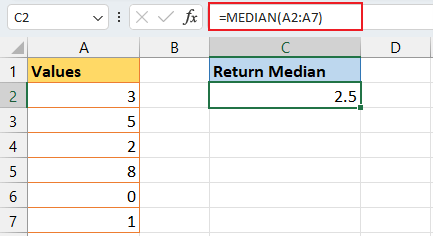Note: If you want to find the median from several discontinuous range, let’s say from A2:A3, A5:A7, please use the formula like this:
``=MEDIAN(A2:A3,A5:A7)``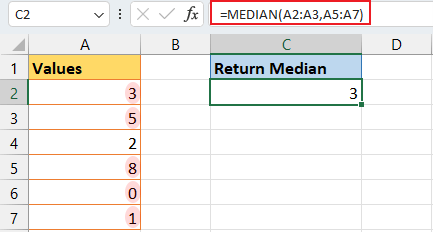##### A Grand Array of Formulas for Complex Calculations - Clicks Away, No Memorization Required

Beyond the MEDIAN function, Excel's AVERAGE function typically finds the dataset's central value. However, it falters when calculating the average of only visible cells. Luckily, Kutools for Excel offers a quick-fix formula -- AVERAGEVISIBLE, that resolves this with just two clicks. Furthermore, it amasses a comprehensive suite of functions and formulas to conquer the myriad of complex calculations encountered in everyday work. Download now and unlock the full potential of Kutools for Excel.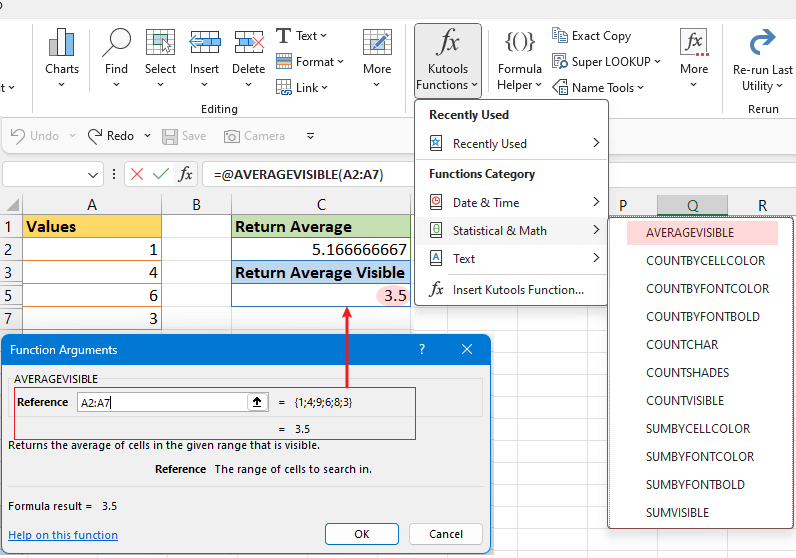#### Example 2: Find the median from a range of cell values excluding zeros

Typically, Excel's MEDIAN function considers zeroes in its calculations. However, if you need to find the median while omitting zero values, you can do so by integrating the IF function with the MEDIAN function.

To calculate the median while excluding zeros in the range A2:A7, enter the following formula in a blank cell and press the Enter key to display the median.

``=MEDIAN(IF(A2:A7>0,A2:A7))``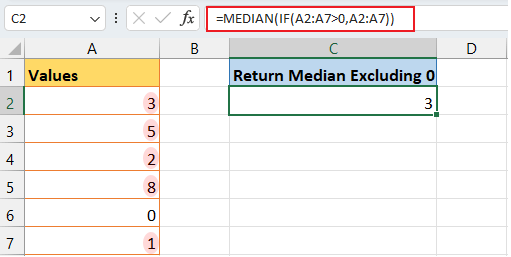Explanation of the formula::
• IF(A2:A7>0, A2:A7): This part of the formula creates an array based on the range A2:A7. For each cell in that range, it checks whether the cell's value is greater than zero. If it is, the value of the cell is included in the array; if not (meaning if the value is zero or negative), it is excluded from the array that will be considered for the median calculation.
• MEDIAN(IF(A2:A7>0,A2:A7)): The MEDIAN function then takes this array (with zeros excluded) and calculates the median value.

The insights shared above examples to calculate median in Excel. I trust this information serves you well. For more game-changing Excel strategies that can elevate your data management, explore further here..

### The Best Office Productivity Tools

#### Kutools for Excel - Helps You To Stand Out From Crowd

 Popular Features: Find, Highlight or Identify Duplicates  |  Delete Blank Rows  |  Combine Columns or Cells without Losing Data  |  Round without Formula ... Super VLookup: Multiple Criteria  |  Multiple Value  |  Across Multi-Sheets  |  Fuzzy Lookup... Adv. Drop-down List: Easy Drop Down List  |  Dependent Drop Down List  |  Multi-select Drop Down List... Column Manager: Add a Specific Number of Columns  |  Move Columns  |  Toggle Visibility Status of Hidden Columns  |  Compare Columns to Select Same & Different Cells ... Featured Features: Grid Focus  |  Design View  |  Big Formula Bar  |  Workbook & Sheet Manager | Resource Library (Auto Text)  |  Date Picker  |  Combine Worksheets  |  Encrypt/Decrypt Cells  |  Send Emails by List  |  Super Filter  |  Special Filter (filter bold/italic/strikethrough...) ... Top 15 Toolsets:  12 Text Tools (Add Text, Remove Characters ...)  |  50+ Chart Types (Gantt Chart ...)  |  40+ Practical Formulas (Calculate age based on birthday ...)  |  19 Insertion Tools (Insert QR Code, Insert Picture from Path ...)  |  12 Conversion Tools (Numbers to Words, Currency Conversion ...)  |  7 Merge & Split Tools (Advanced Combine Rows, Split Excel Cells ...)  |  ... and more

Kutools for Excel Boasts Over 300 Features, Ensuring That What You Need is Just A Click Away...#### Office Tab - Enable Tabbed Reading and Editing in Microsoft Office (include Excel)

• One second to switch between dozens of open documents!
• Reduce hundreds of mouse clicks for you every day, say goodbye to mouse hand.
• Increases your productivity by 50% when viewing and editing multiple documents.
• Brings Efficient Tabs to Office (include Excel), Just Like Chrome, Edge and Firefox.No ratings yet. Be the first to rate!
This comment was minimized by the moderator on the site
This is really useful. I've had some success with this method but tried to extend it a little without any joy.

I am wanting to vary the criteria if cell AB6 is blank. The following is successful whether ISBLANK is true or false:

{=IF(ISBLANK(\$AB\$6),MEDIAN(\$O:\$O),MEDIAN(IF(\$A:\$A=\$AB\$6,IF(\$S:\$S<>"X",\$O:\$O))))}

I also want to check for "X" in col S for both sides of the argument, but the following formula returns 0 in error when ISBLANK = TRUE, but still functions as expected when ISBLANK = FALSE.

{=IF(ISBLANK(\$AB\$6),MEDIAN(IF(\$S:\$S<>"X",\$O:\$O)),MEDIAN(IF(\$A:\$A=\$AB\$6,IF(\$S:\$S<>"X",\$O:\$O))))}

Is there a limitation that stops me using an array formula for both TRUE or FALSE? Have I got something wrong in the formula maybe? Any suggestions?
This comment was minimized by the moderator on the site
It was a nice article. It helped me. Please check one thing in the section Calculate Median Excluding Zero In A Range Below there in the first line the word excluding is to be replaced by including
There are no comments posted here yet